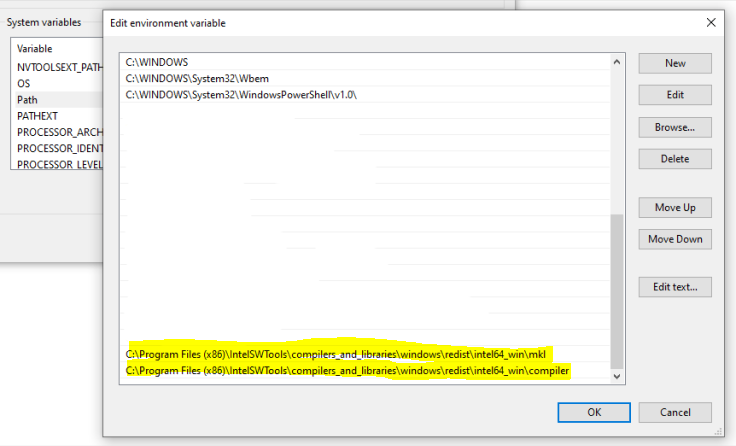# R with Intel MKL for Windows

R for Windows that is downloaded from CRAN uses the reference BLAS and LAPACK implementations for linear algebra operations. The reference implementations are very stable and compatible across platforms, but are not optimized for performance. By switching to a highly optimized BLAS library such as the Intel MKL, you could see significant performance improvements for linear algebra computations. In the following I will show how to replace the standard BLAS and LAPACK implementations with the Intel MKL.

I assume that you already have Intel MKL installed on your Windows computer, otherwise installation instructions can be found here.

In the first step, run the R benchmark script 1-r-benchmark-25.R with the reference BLAS and LAPACK such that you can later compare with the Intel MKL. For example, on my computer the benchmark script reported

R Benchmark 2.5
===============
Number of times each test is run__________________________:  3

I. Matrix calculation
---------------------
Creation, transp., deformation of a 2500x2500 matrix (sec):  0.689999999999979
2400x2400 normal distributed random matrix ^1000____ (sec):  0.796666666666662
Sorting of 7,000,000 random values__________________ (sec):  0.716666666666678
2800x2800 cross-product matrix (b = a' * a)_________ (sec):  13.4233333333333
Linear regr. over a 3000x3000 matrix (c = a \ b')___ (sec):  6.40333333333335
--------------------------------------------
Trimmed geom. mean (2 extremes eliminated):  1.54051756267069

II. Matrix functions
--------------------
FFT over 2,400,000 random values____________________ (sec):  0.270000000000001
Eigenvalues of a 640x640 random matrix______________ (sec):  0.676666666666658
Determinant of a 2500x2500 random matrix____________ (sec):  3.61666666666667
Cholesky decomposition of a 3000x3000 matrix________ (sec):  5.39666666666669
Inverse of a 1600x1600 random matrix________________ (sec):  3.01999999999998
--------------------------------------------
Trimmed geom. mean (2 extremes eliminated):  1.9478854034592

III. Programmation
------------------
3,500,000 Fibonacci numbers calculation (vector calc)(sec):  0.586666666666626
Creation of a 3000x3000 Hilbert matrix (matrix calc) (sec):  0.213333333333367
Grand common divisors of 400,000 pairs (recursion)__ (sec):  0.220000000000027
Creation of a 500x500 Toeplitz matrix (loops)_______ (sec):  0.0433333333333318
Escoufier's method on a 45x45 matrix (mixed)________ (sec):  0.280000000000086
--------------------------------------------
Trimmed geom. mean (2 extremes eliminated):  0.235982506922657

Total time for all 15 tests_________________________ (sec):  36.3533333333334
Overall mean (sum of I, II and III trimmed means/3)_ (sec):  0.891326097331175
--- End of test ---

Then, go to the folder where the R dll’s are installed and rename the BLAS and LAPACK dll’s. On my computer I executed the following commands from a command prompt as Administrator

cd "C:\Program Files\R\R-4.0.0\bin\x64"
rename Rblas.dll Rblas.dll.orig
rename Rlapack.dll Rlapack.dll.orig

Next, go to the folder where the Intel MKL dll’s are installed and copy the files mkl_rt.dll and mkl_intel_thread.dll to the folder where the R dll’s are installed. On my computer I executed the following, again from a command prompt as Administrator

cd "C:\Program Files (x86)\IntelSWTools\compilers_and_libraries\windows\redist\intel64_win\mkl"
copy mkl_rt.dll "C:\Program Files\R\R-4.0.0\bin\x64\Rblas.dll"
copy mkl_rt.dll "C:\Program Files\R\R-4.0.0\bin\x64\Rlapack.dll"
copy mkl_intel_thread.dll "C:\Program Files\R\R-4.0.0\bin\x64"

Finally, add the folder where the Intel MKL dll’s are installed and another Intel MKL folder to the PATH. On my computer I added the highlighted pathsNow, you should be able to run the R benchmark script 1-r-benchmark-25.R with the Intel MKL BLAS and LAPACK. On my computer the benchmark script this time reported

R Benchmark 2.5
===============
Number of times each test is run__________________________:  3

I. Matrix calculation
---------------------
Creation, transp., deformation of a 2500x2500 matrix (sec):  0.62
2400x2400 normal distributed random matrix ^1000____ (sec):  0.743333333333334
Sorting of 7,000,000 random values__________________ (sec):  0.673333333333333
2800x2800 cross-product matrix (b = a' * a)_________ (sec):  0.183333333333332
Linear regr. over a 3000x3000 matrix (c = a \ b')___ (sec):  0.100000000000001
--------------------------------------------
Trimmed geom. mean (2 extremes eliminated):  0.424574994841722

II. Matrix functions
--------------------
FFT over 2,400,000 random values____________________ (sec):  0.233333333333333
Eigenvalues of a 640x640 random matrix______________ (sec):  0.206666666666666
Determinant of a 2500x2500 random matrix____________ (sec):  0.090000000000001
Cholesky decomposition of a 3000x3000 matrix________ (sec):  0.0899999999999999
Inverse of a 1600x1600 random matrix________________ (sec):  0.12
--------------------------------------------
Trimmed geom. mean (2 extremes eliminated):  0.130686701541752

III. Programmation
------------------
3,500,000 Fibonacci numbers calculation (vector calc)(sec):  0.529999999999999
Creation of a 3000x3000 Hilbert matrix (matrix calc) (sec):  0.193333333333335
Grand common divisors of 400,000 pairs (recursion)__ (sec):  0.203333333333331
Creation of a 500x500 Toeplitz matrix (loops)_______ (sec):  0.0333333333333338
Escoufier's method on a 45x45 matrix (mixed)________ (sec):  0.25
--------------------------------------------
Trimmed geom. mean (2 extremes eliminated):  0.214199495002168

Total time for all 15 tests_________________________ (sec):  4.27
Overall mean (sum of I, II and III trimmed means/3)_ (sec):  0.228210041938561
--- End of test ---

As can be seen from the Benchmark results, R with the Intel MKL is significantly faster, in my example runs based on the total time of the benchmark script about 8 times faster than R with the default BLAS and LAPACK.